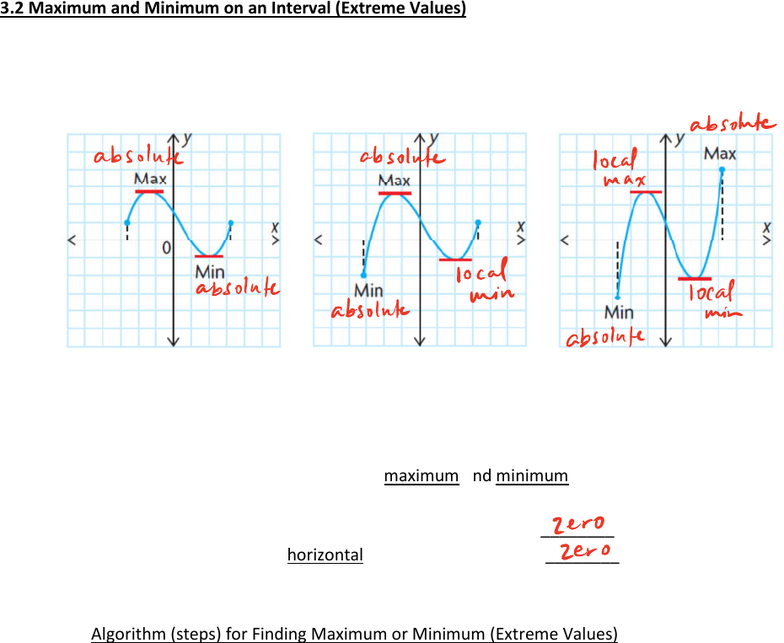# MAT135H1 Lecture 8: 3.2 Max and Min Values on an Interval

19 views3 pages3.2 Maximum and Minimum on an Interval (Extreme Values)
Calculus can be used to determine the maximum and minimum values of a function on an interval
The derivative at the maximum and minimum is equal to _________________
! i.e. the tangent is horizontal (slope of the tangent is _______________ )
Algorithm (steps) for Finding Maximum or Minimum (Extreme Values)
! For
()fx
on an interval [a, b]
1) Find the derivative,
'( )fx
2) Find all points in the interval [a, b] where
'( ) 0fx=
3) Evaluate
()fx
at the endpoints of the function [a, b], and points where
'( ) 0fx=
4) Compare the value is step 3:
o the largest value is the max value of
()fx
on the interval
o the smallest value is the min value of
()fx
on the interval
absolute
absolute absolute local
max
local
absolute min local
absolute min
absolute
zero
zero
Unlock document

This preview shows page 1 of the document.
Unlock all 3 pages and 3 million more documents.